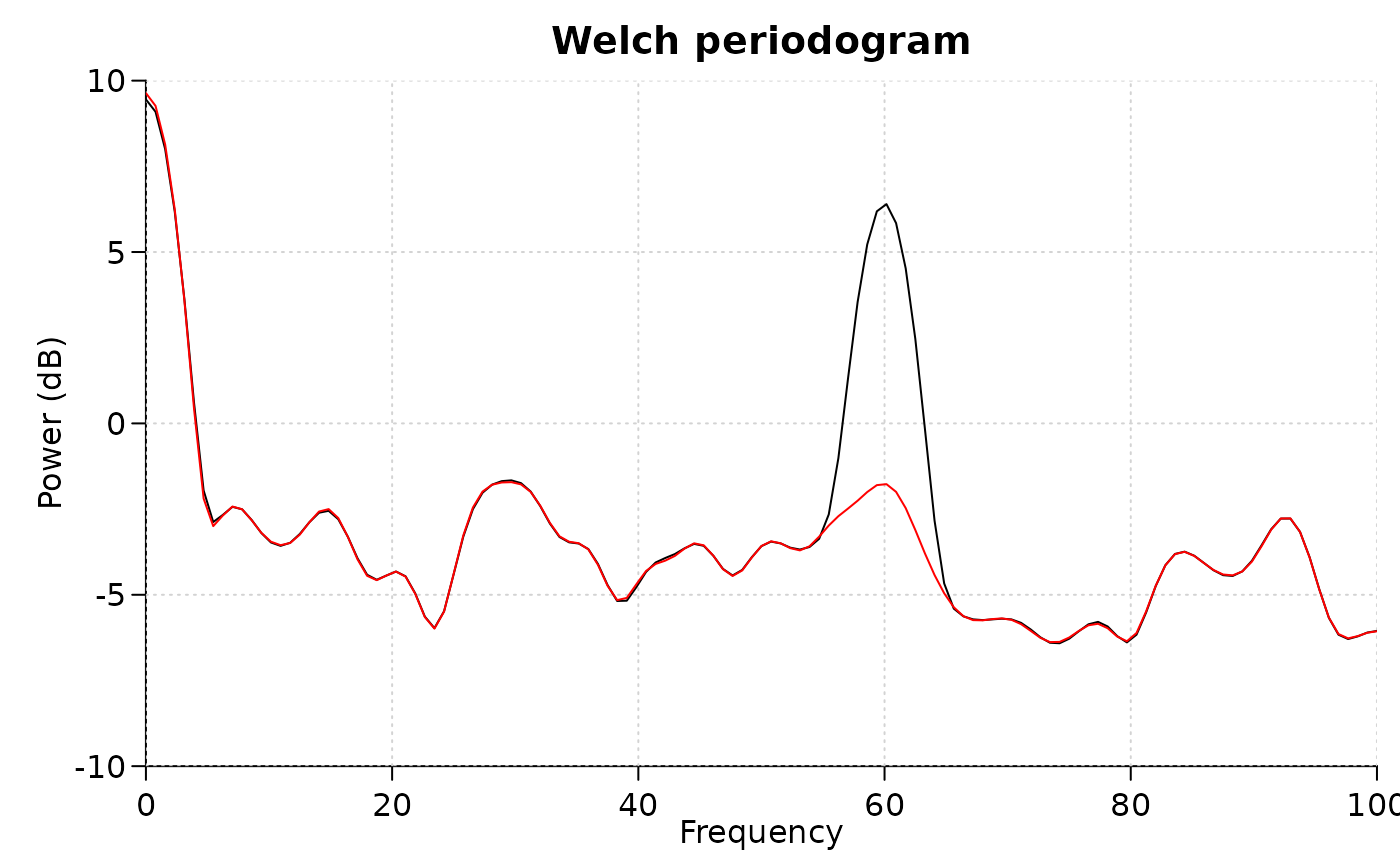Apply 'Notch' filter

## Usage

notch_filter(
s,
sample_rate,
lb = c(59, 118, 178),
ub = c(61, 122, 182),
domain = 1
)

## Arguments

s

numerical vector if domain=1 (voltage signals), or complex vector if domain=0

sample_rate

sample rate

lb

filter lower bound of the frequencies to remove

ub

filter upper bound of the frequencies to remove; shares the same length as lb

domain

1 if the input signal is in the time domain, 0 if it is in the frequency domain

## Value

filtered signal in time domain (real numerical vector)

## Details

Mainly used to remove electrical line frequencies at 60, 120, and 180 Hz.

## Examples



time <- seq(0, 3, 0.005)
s <- sin(120 * pi * time) + rnorm(length(time))

# Welch periodogram shows a peak at 60Hz
pwelch(s, 200, plot = 1, log = "y")

# notch filter to remove 60Hz
s1 <- notch_filter(s, 200, lb = 59, ub = 61)
pwelch(s1, 200, plot = 2, log = "y", col = "red")